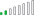cancel
Showing results for
Did you mean:New Member

## Using a calculated measure by filtered date in future months

I am trying to use a calculated measure from the last date in my dataset for future months to forecast. Because I am filtering the value based on a specific date, it does not recognize it when I try to use this value for future months.

I flagged the month, hard coded for now, then identified the value at that date.

History Stop Date = IF('*Calendar'[Date]=DATE(2022,1,1),"Y","N")
Forecast Start = CALCULATE([Forecast],'*Calendar'[History Stop Date]="Y")

When I try to use the Forecast Start in calculations post 1/1/2021, it returns blanks. How can I grab this value to use in future calculations? Thanks!
2 REPLIES 2Community Support

Hi  @jllane ,

You can change [Forecast Start ] to the following form:

``````Forecast Start =
CALCULATE(MAX('Calendar'[Forecast]),FILTER(ALL('Calendar'),'Calendar'[History Stop Date]="Y"))``````

Best Regards,

Liu Yang

If this post helps, then please consider Accept it as the solution to help the other members find it more quickly.Resolver II

Hi @jllane ,

Does the calender table has any relationship (connected) with the fact table which [forcast] is referring to?

Is there any value for [forcat] at 1/1/2021?

Thanks!